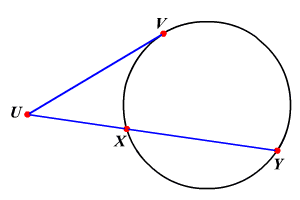# Intersecting Secant-Tangent Theorem

If a tangent segment and a secant segment are drawn to a circle from an exterior point, then the square of the measure of the tangent segment is equal to the product of the measures of the secant segment and its external secant segment.In the circle, $\stackrel{¯}{UV}$ is a tangent and $\stackrel{¯}{UY}$ is a secant. They intersect at point $U$ .

So, $U{V}^{2}=UX\cdot UY$ .

Example :

In the circle shown, if $UX=8$ and $XY=10$ , then find the length of $UV$ .

Substitute.

$\begin{array}{l}U{V}^{2}=UX\cdot UY\\ \text{\hspace{0.17em}}\text{\hspace{0.17em}}\text{\hspace{0.17em}}\text{\hspace{0.17em}}\text{\hspace{0.17em}}\text{\hspace{0.17em}}\text{\hspace{0.17em}}\text{\hspace{0.17em}}\text{\hspace{0.17em}}\text{\hspace{0.17em}}\text{\hspace{0.17em}}=\left(UX\right)\left(UX+XY\right)\\ \text{\hspace{0.17em}}\text{\hspace{0.17em}}\text{\hspace{0.17em}}\text{\hspace{0.17em}}\text{\hspace{0.17em}}\text{\hspace{0.17em}}\text{\hspace{0.17em}}\text{\hspace{0.17em}}\text{\hspace{0.17em}}\text{\hspace{0.17em}}\text{\hspace{0.17em}}=\left(8\right)\left(8+10\right)\\ \text{\hspace{0.17em}}\text{\hspace{0.17em}}\text{\hspace{0.17em}}\text{\hspace{0.17em}}\text{\hspace{0.17em}}\text{\hspace{0.17em}}\text{\hspace{0.17em}}\text{\hspace{0.17em}}\text{\hspace{0.17em}}\text{\hspace{0.17em}}\text{\hspace{0.17em}}=\left(8\right)\left(18\right)\\ \text{\hspace{0.17em}}\text{\hspace{0.17em}}\text{\hspace{0.17em}}\text{\hspace{0.17em}}\text{\hspace{0.17em}}\text{\hspace{0.17em}}\text{\hspace{0.17em}}\text{\hspace{0.17em}}\text{\hspace{0.17em}}\text{\hspace{0.17em}}\text{\hspace{0.17em}}=144\end{array}$

Take the square root on each side.

$\begin{array}{l}U{V}^{2}=\sqrt{144}\\ \text{\hspace{0.17em}}\text{\hspace{0.17em}}UV=12\end{array}$